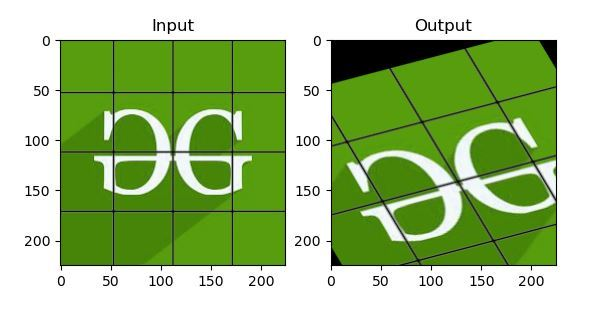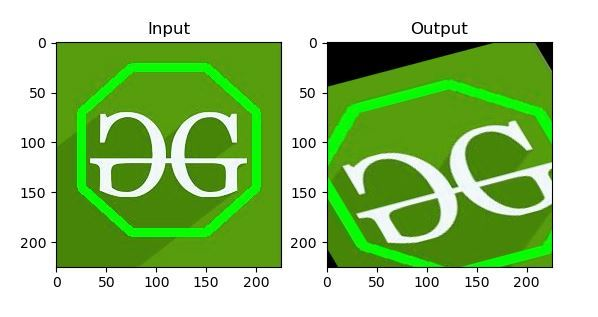# Python OpenCV – Affine Transformation

OpenCV is the huge open-source library for computer vision, machine learning, and image processing and now it plays a major role in real-time operation which is very important in today’s systems. By using it, one can process images and videos to identify objects, faces, or even the handwriting of a human. When it integrated with various libraries, such as Numpuy, Python is capable of processing the OpenCV array structure for analysis.

## Affine Transformation

In Affine transformation, all parallel lines in the original image will still be parallel in the output image. To find the transformation matrix, we need three points from input image and their corresponding locations in the output image. Then cv2.getAffineTransform will create a 2×3 matrix which is to be passed to cv2.warpAffine.

cv2.getAffineTransform method:

Syntax: cv2.getPerspectiveTransform(src, dst)

Parameters:
src: Coordinates of quadrangle vertices in the source image.
dst: Coordinates of the corresponding quadrangle vertices in the destination image.

cv2.warpAffine method:

Syntax: cv2.warpAffine(src, M, dsize, dst, flags, borderMode, borderValue)

Parameters:
src: input image.
dst: output image that has the size dsize and the same type as src.
M: transformation matrix.
dsize: size of the output image.
flags: combination of interpolation methods (see resize() ) and the optional flag
WARP_INVERSE_MAP that means that M is the inverse transformation (dst->src).
borderMode: pixel extrapolation method; when borderMode=BORDER_TRANSPARENT, it means that the pixels in the destination image corresponding to the “outliers” in the source image are not modified by the function.
borderValue: value used in case of a constant border; by default, it is 0.

Example 1:

 `import` `cv2 ` `import` `numpy as np ` `from` `matplotlib ``import` `pyplot as plt ` ` `  ` `  `img ``=` `cv2.imread(``'food.jpeg'``) ` `rows, cols, ch ``=` `img.shape ` ` `  `pts1 ``=` `np.float32([[``50``, ``50``], ` `                   ``[``200``, ``50``],  ` `                   ``[``50``, ``200``]]) ` ` `  `pts2 ``=` `np.float32([[``10``, ``100``], ` `                   ``[``200``, ``50``],  ` `                   ``[``100``, ``250``]]) ` ` `  `M ``=` `cv2.getAffineTransform(pts1, pts2) ` `dst ``=` `cv2.warpAffine(img, M, (cols, rows)) ` ` `  `plt.subplot(``121``) ` `plt.imshow(img) ` `plt.title(``'Input'``) ` ` `  `plt.subplot(``122``) ` `plt.imshow(dst) ` `plt.title(``'Output'``) ` ` `  `plt.show() ` ` `  `# Displaying the image ` `while``(``1``): ` `     `  `    ``cv2.imshow(``'image'``, img) ` `    ``if` `cv2.waitKey(``20``) & ``0xFF` `=``=` `27``: ` `        ``break` `         `  `cv2.destroyAllWindows() `

Output:Example 2:

 `import` `cv2 ` `import` `numpy as np ` `from` `matplotlib ``import` `pyplot as plt ` ` `  ` `  `img ``=` `cv2.imread(``'food.jpeg'``) ` `rows, cols, ch ``=` `img.shape ` ` `  `pts1 ``=` `np.float32([[``50``, ``50``],  ` `                   ``[``200``, ``50``], ` `                   ``[``50``, ``200``]]) ` ` `  `pts2 ``=` `np.float32([[``10``, ``100``], ` `                   ``[``200``, ``50``],  ` `                   ``[``100``, ``250``]]) ` ` `  `M ``=` `cv2.getAffineTransform(pts1, pts2) ` `dst ``=` `cv2.warpAffine(img, M, (cols, rows)) ` ` `  `plt.subplot(``121``) ` `plt.imshow(img) ` `plt.title(``'Input'``) ` ` `  `plt.subplot(``122``) ` `plt.imshow(dst) ` `plt.title(``'Output'``) ` `plt.show() ` ` `  `# Displaying the image ` `while``(``1``): ` `     `  `    ``cv2.imshow(``'image'``, img) ` `    ``if` `cv2.waitKey(``20``) & ``0xFF` `=``=` `27``: ` `        ``break` `         `  `cv2.destroyAllWindows() `

Output:Attention geek! Strengthen your foundations with the Python Programming Foundation Course and learn the basics.

To begin with, your interview preparations Enhance your Data Structures concepts with the Python DS Course.

My Personal Notes arrow_drop_upCheck out this Author's contributed articles.

If you like GeeksforGeeks and would like to contribute, you can also write an article using contribute.geeksforgeeks.org or mail your article to contribute@geeksforgeeks.org. See your article appearing on the GeeksforGeeks main page and help other Geeks.

Please Improve this article if you find anything incorrect by clicking on the "Improve Article" button below.

Article Tags :

2

Please write to us at contribute@geeksforgeeks.org to report any issue with the above content.